# DRConv: Ambient Vision proposes region-aware dynamic convolution, multitasking performance improvement | CVPR 2020

Posted Jun 5, 20207 min read

The paper proposes DRConv, which combines the idea of local sharing well and maintains translation invariance. It contains two key structures. From the experimental results, DRConv meets the expectations of the design and has a good performance improvement on multiple tasks.

Source:Xiaofei's algorithm engineering notes

Paper:Dynamic Region-Aware Convolution# Introduction

The current mainstream convolution operations all share weights in the spatial domain, and if you want to get more rich information, you can only achieve it by increasing the number of convolutions, which not only inefficient calculation, but also bring network optimization difficulties. Unlike mainstream convolution, local conv uses different weights at different pixel positions, which can efficiently extract rich information, mainly used in the field of face recognition, but local conv will not only bring parameters related to the size of the feature map Volume, it will also destroy translation invariance.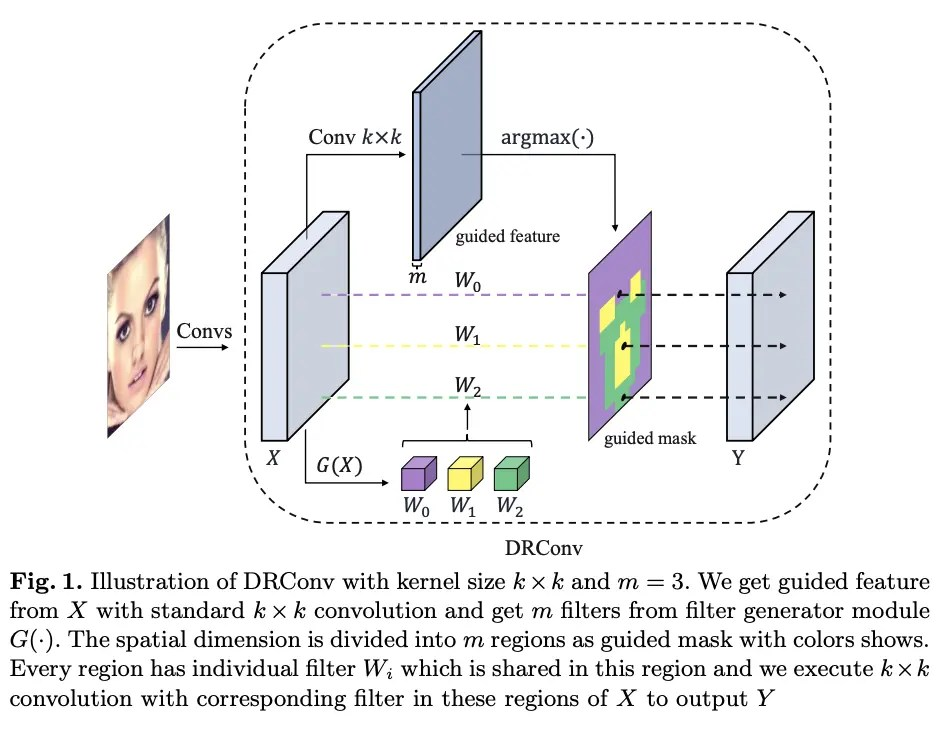Considering the advantages and disadvantages of the above two convolutions, the paper proposes DRConv(Dynamic Region-Aware Convolution). The structure of DRConv is shown in Fig. 1. First, the guided feature is generated by standard convolution, and the spatial dimension is divided into multiple regions according to the guided feature , The convolution kernel generation module $G(\cdot)$dynamically generates the convolution kernel corresponding to each area according to the input picture. DRConv can learnably match different convolution kernels for different pixel positions, which not only has a strong feature expression ability, but also maintains translation invariance. Because the convolution kernel is dynamically generated, it can reduce a large number of parameters compared to local conv, and the overall calculation amount is almost consistent with the standard convolution.
The main contributions of the paper are as follows:

• Proposed DRConv, which not only has strong semantic expression ability, but also maintains translation invariance well.
• Ingeniously designed the back propagation of the learned guided mask, clear the region-sharing-pattern, and update according to the gradient of the loss function return.
• With simple replacement, DRConv can achieve very good performance in multiple tasks such as image classification, face recognition, target detection and semantic segmentation.

# Our Apporach

### Dynamic Region-Aware Convolution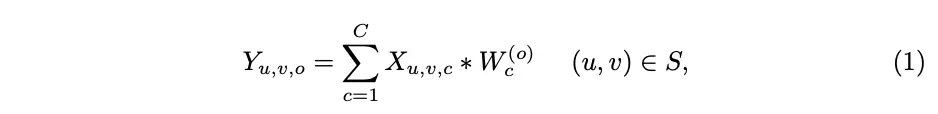For standard convolution, define the input $X\in \mathbb{R}^{U\times V\times C}$, and the spatial dimension $S\in \mathbb{R}^{U\ times V}$, output $Y\in \mathbb{R}^{U\times V\times O}$, weight $W\in \mathbb{R}^C$, each output The calculation of each channel is as formula 1, $*$is a two-dimensional convolution operation.For the basic local conv, define the non-shared weight $W\in \mathbb{R}^{U\times V\times C}$, and each output channel is calculated as formula 2, where $W_ {u,v,c}^{(o)}$represents an independent non-shared convolution kernel at position $(u,v)$, that is, when the convolution moves on the feature map, different convolution kernels are replaced each time .Combined with the above formula, define guided mask$M={S_0, \cdots,S_{m-1}}$to represent $m$regions divided by spatial dimensions, $M$according to the characteristics of the input picture For extraction, each region $S_t(t\in [0, m-1])$uses only one shared convolution kernel. Define the convolution kernel set $W=[W_0,\cdots,W_{m-1}]$, corresponding to the convolution kernel $W_t \in \mathbb{R}^C$In the area $S_t$. The calculation of each output channel is as shown in Formula 3, that is, when the convolution moves on the feature map, the corresponding convolution kernel is replaced every time according to the guided mask.
As you can see from the above description, DRConv contains two main parts:

• Use a learnable guided mask to divide the spatial dimension into multiple areas. As shown in Figure 1, pixels of the same color in the guided mask are classified as the same area. From a semantic point of view, semantically similar features are unified region.
• For each shared area, a convolution kernel generation module is used to generate a customized convolution kernel to perform conventional 2D convolution operations. The customized convolution kernel can be automatically adjusted according to the important characteristics of the input picture.

As an important part of DRConv, the guided mask determines the distribution of the convolution kernel in the spatial dimension. This module is guided by the loss function to optimize, so that it can adapt to the change of the input spatial information and thus change the distribution of the convolution kernel.For $k\times k$DRConv containing $m$channels, define $F$as a guided feature, $M$as a guided mask, and calculate the value of $(u,v)$for each position on $M$As in formula 4, the function $argmax(\cdot)$outputs the subscript of the maximum value, $F_{u,v}$is the guided feature vector at position $(u,v)$, so the $M$The value is $[0, m-1]$, which is used to indicate the convolution subscript corresponding to the position.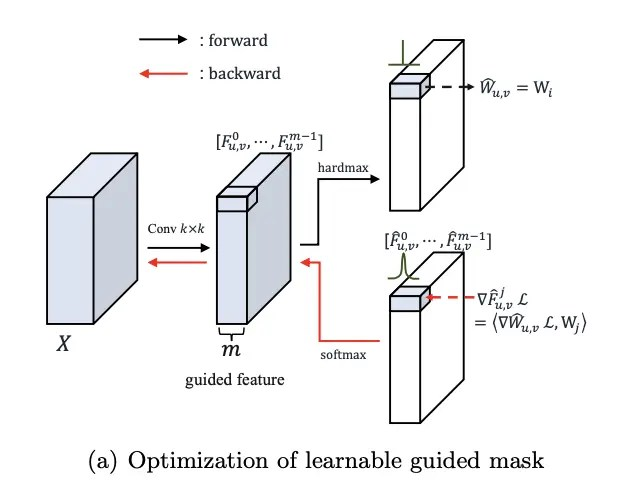In order to make the guided mask learnable, the gradient used to generate the weight of the guided feature must be obtained, but the gradient of the guided feature cannot be calculated due to the use of $argmax(\cdot)$, so the paper designed a similar gradient.

• ##### Forward propagation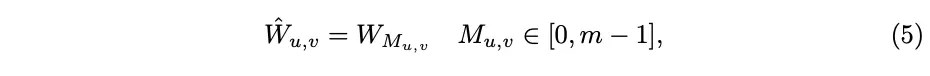Get the guided mask according to formula 4, and get the convolution kernel $(u,v)$for each position according to formula 5. $\tilde{W}_{u,v}$, where $W_{M_ {u,v}}$is one of the convolution kernel sets $[W_0, \cdots, W_{m-1}]$generated by $G(\cdot)$, $M _{u,v}$is the channel index where the guided feature has the highest value at position $(u,v)$. In this way, the relationship between $m$convolution kernels and all positions is divided into spatial pixels. For $m$groups. Pixels that use the same convolution kernel contain similar context information, mainly due to the standard convolution with translation invariance that passes this information to the guided feature.

• ##### Backward propagationIn order to get the gradient back, first use $\hat{F}$to replace the one-hot representation of the guided mask. The calculation is as shown in Equation 6, $softmax(\cdot)$is performed on the channel dimension, and the expected $\hat{F}_{u,v}^j$can be as close to 0 and 1 as possible, so that one of the $\hat{F}_{u,v}^j$and guided mask is one-hot The representation will be very similar. Equation 5 can be seen as a one-hot representation of the convolution kernel set $[W_0,\cdots,W_{m-1}]$multiplied by $M_{u,v}$, Replaced here with $\hat{F}_{u,v}^j$.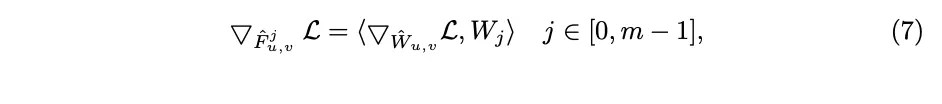The gradient of $\hat{F}_{u,v}^j$is calculated as formula 7, $\langle, \rangle$is the dot product, $\bigtriangledown_{\cdot} \ Mathcal{L}$represents the gradient of the guided mask corresponding to the loss function. As shown in Figure a, Equation 7 is similar to the back propagation of Equation 5.Equation 8 is the back propagation of Equation 6, and $\odot$is an element-by-element multiplication. If no special back propagation is designed, SGD will not be able to optimize the relevant parameters because the function $argmax(\cdot)$It is unguided. Therefore, $softmax(\cdot)$is used to approximate $argmax(\cdot)$, and the gradient is passed back to the guided feature through the replacement function. The guided mask is learnable.

### Dynamic Filter:Filter generator module

In DRConv, a convolution kernel generation module is used to generate convolution kernels in different regions. Due to the different characteristics of different pictures, the convolution kernel shared between pictures cannot efficiently extract its unique features, and needs customized features to Focus on the characteristics of different pictures.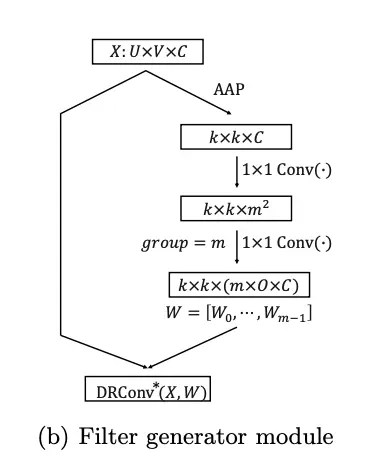Define the input $X\in \mathbb{R}^{U\times V\times C}$, including two layers of convolution kernel generation module $G(\cdot)$, $m$Convolutions $W=[W_0,\cdots,W_{m-1}]$, each convolution is only used for the region $R_t$. As shown in Figure b, to obtain $m$convolutions of $k\times k$, first use adaptive average pooling to downsample $X$to $k\times k$, and then use two consecutive $1\times 1$convolution, the first one uses $sigmoid(\cdot)$for activation, and the second one sets $group=m$, without activation. The convolution kernel generation module can enhance the network's ability to acquire different image characteristics. Since the convolution kernel is generated according to the input features, the focus of each convolution kernel can be automatically adjusted according to the input characteristics.

# Experiments

### Classification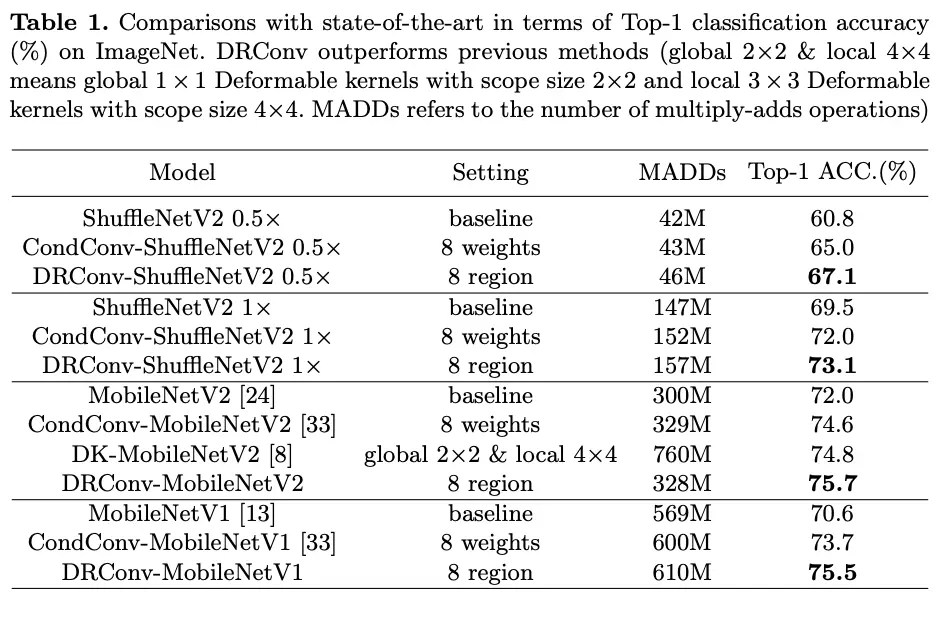### Face Recognition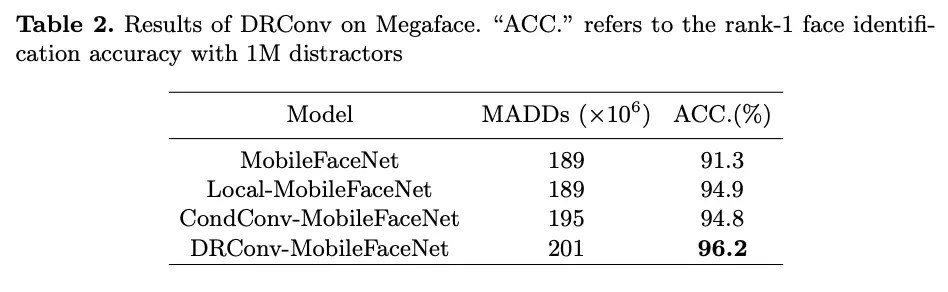### COCO Object Detection and Segmentation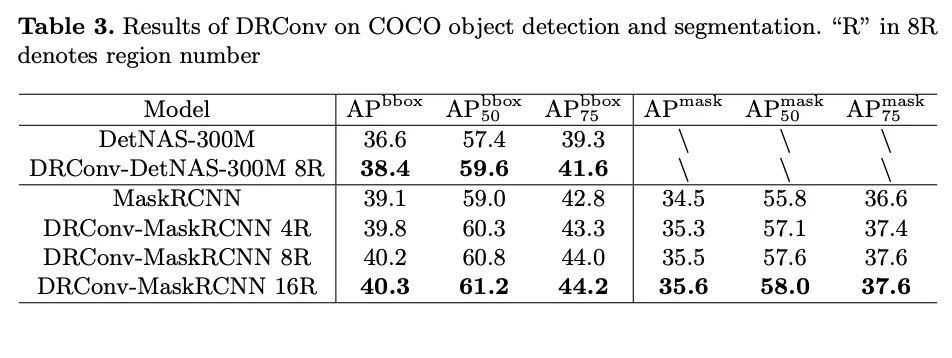# Ablation Study

### Visualization of dynamic guided mask### Different model size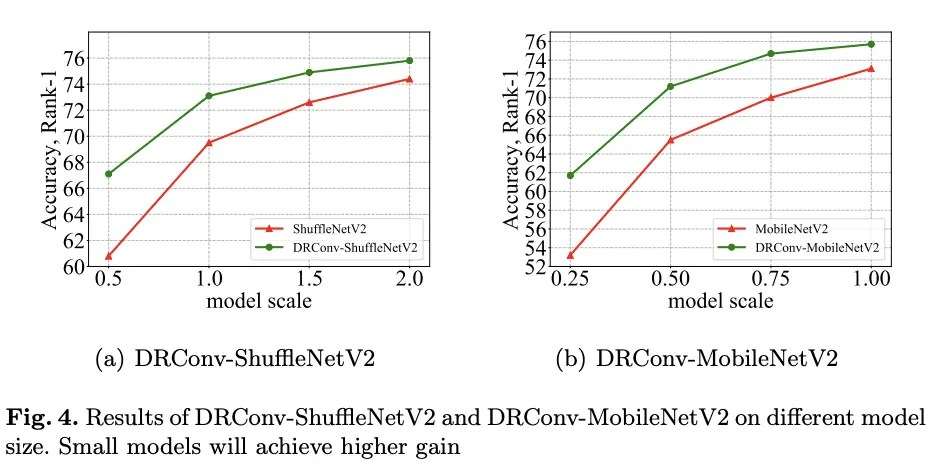### Different region number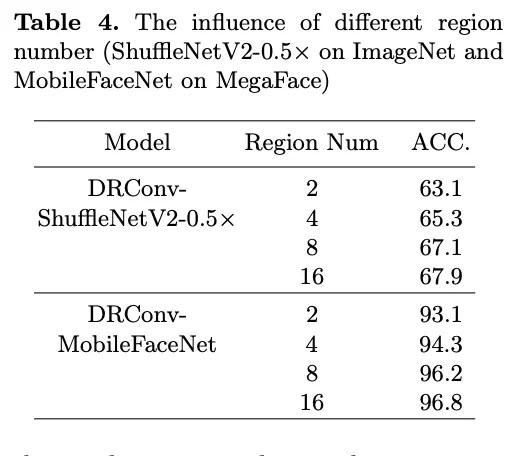### Different spatial size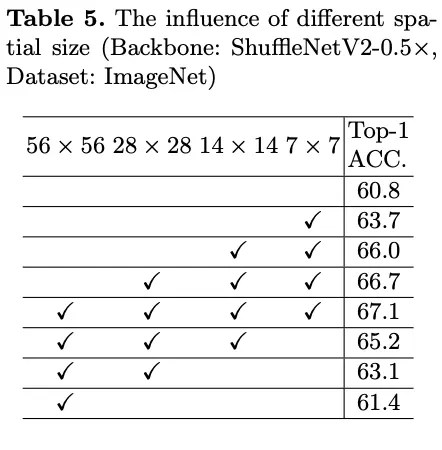# CONCLUSION

The paper proposes DRConv, which combines the idea of local sharing well and maintains translation invariance. It contains two key structures. First, the guided mask is used to divide the pixels in the feature map into different regions, and secondly, the convolution kernel generation module is used to dynamically generate Convolution kernel corresponding to the region. From the experimental results, DRConv meets the design expectations, especially the visualized result of the guided mask in Figure 3, which has a good performance improvement on multiple tasks.